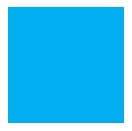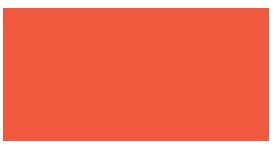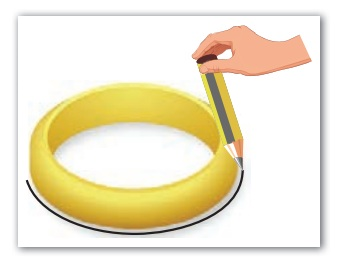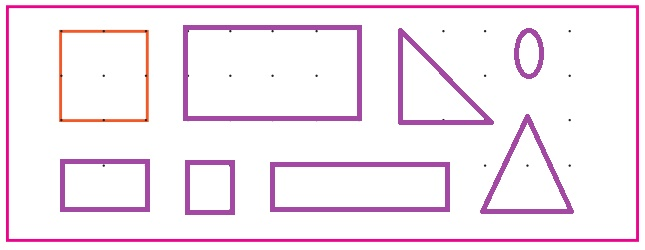Home | | Maths 3rd Std | Construction of 2D shapes

# Construction of 2D shapes

Let us understand the properties of 2D shapes

Construction of 2D shapes

Let us understand the properties of 2D shapes.

SquareLet us know: A Square has four sides. All the four sides are equal.

Let us make a square by folding a paper by following the given steps.

Step 1: Take a paperStep 2: Fold the paper as shown in the figure.

Shade the extra portion in the bottom with red colour. Red coloured portion will be rectangular in shape. Tear it off and keep it aside. Open up the triangle. What do you observe? You could see a square.The crease in the middle of the square is called the 'Diagonal of the square'.

You can note that the diagonal divides the square into two trianlges.

Try this

Can you find the other diagonal of the square by folding it the other way? If so how many diagonals can you find for a square?.Teacher's note: Teacher can guide the children to do this paper folding activity.

Observe the number of sides and corners of a square.

So, a square has four sides, four corners and two diagonals.

Think

Are all the sides of a square equal

Are they equal?

We know from square shape

we shall summarize the properties of a square as follows* Square has four sides.

* All the four sides are equal.

* Square has four corners.

* Square has two diagonals.

* The two diagonals are equal.

Rectangle

Step 1: Take the rectangular piece which was kept aside. Observe its sides.Let us know: Opposite sides are equal

Fold the opposite sides of the rectangle. What do you observe? The sides coincide.Now we get opposite sides equal. Hence in a rectangle, opposite sides are equal.

Fold the opposite corners as we did in the square. Observe the crease. It shows the diagonal of the rectangle.Let us know: Diagonals are equal in rectangle.

We know from rectangle shape

The properties of a rectangle are as follows* Rectangle has four sides.

* Two opposite sides are equal.

* Rectangle has four corners.

* Rectangle has two diagonals.

* Two diagonals are equal.

Rectangle

Fold the square along any of these diagonals to form a triangle.Observe the Sides and corners of the rectangle.

A triangle has three sides and three corners.

Cut the paper and make triangles of different kind.

Observe the length of the sides of the triangle. length of the sides. Let the children explore the names of different sides of the triangles.

Try This: How many triangles can be made out of this square paper?

Fold the square along any of diagonals to form a triangle.

Let us knowTeacher’s note: Facilitate the children to explore the properties of shapes in various aspects.

Circle

Let us know: Circle is a closed curveDraw a circle using pencil and Bangle.

Step 1: Place a bangle on the paper as shown in figure.Step 2: Trace the outline of the bangle with a curved line with the pencil until you reach the starting point, we get a circle.Now, we get a circle

On observing the circle drawn we shall write the properties of it as follows.

* Circle has no sides.

* Circle has no corners.

* Circle has a center point.Activity 1

Write the names of few objects in everyday use and mention their geometrical shapes. Example, table− cuboid

wheels of a bike − Circle – Cylinder

ball – sphere

pencil - cylinder

clock faces − Circle

dinner plates − Circle

chess boards − Square

slices of pizza − Triangle

chapter book covers − Rectangle

cell phones – Rectangle - cuboid

Practice

1) Triangle has Three corners.

2) Four sides of a square are Equal.

3) Circle has No sides.

4) Rectangle has Two diagonals.

5) Opposite sides of a rectangle are Equal.

6) Circle has One centre point.

Plane Surface

Surface of few objects like walls, floors papers and top of a table are flat. Flat surfaces are otherwise called as plane surfaces or planes. Cubes and cuboids have flat surfaces.Curved Surface

Surfaces of few objects such as ball, flowerwase, pot are curved. Cone, Cylinder and sphere have curved surfaces.Activity 2

Draw all the 2d shapes in the given dot grid. One is done for you.

A geoboard is a mathematical manipulative board.Join the dots in the grid using curved lines to make designs of your choice. one is done for you.Teacher’s note : Teacher can lead the children to make the shapes drawn by them in the dot grid by using rubber band in the Geo board drawn by them in the dot grid

Activity 3

We can see many things around us have straight lines and curved lines.

Draw any 5 shapes and put a tick in the given boxes to indicate the type of the lines found in them.Teacher’s note : Teacher can discuss about the types of lines found in objects in everyday use and enable the children to draw them in above tabular column.

Practice

Put a tick mark in the appropriate columns.Tags : Geometry | Term 1 Chapter 1 | 3rd Maths , 3rd Maths : Term 1 Unit 1 : Geometry
Study Material, Lecturing Notes, Assignment, Reference, Wiki description explanation, brief detail
3rd Maths : Term 1 Unit 1 : Geometry : Construction of 2D shapes | Geometry | Term 1 Chapter 1 | 3rd Maths